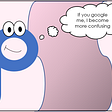# A Visual Intuition of Bayes Rule

A question from Quora made me think about the intuition of Bayes theorem, so I tried to work out a visual intuition from one of the scenarios mentioned in the answers.

## Problem:

Let's say, your friend is trying to convince you that being rich does not make someone happy by quoting a reputed study, which says only 10% of happy people are rich. You being a logical person, you know that the actual statistic that would make sense here is the percentage of rich people that are happy and not the other way around. So you set out to find it out using Bayes rule.

## Bayes rule:

Bayes rule is often used to find the reverse probabilities of a known conditional probability. In our case, we would like to find P(Happy|Rich) from the known value P(Rich|Happy),

`P(Happy|Rich) = (P(Happy) X P(Rich|Happy))/ P(Rich)`

Let us try to have a visual intuition of the above equation by considering that the probability of someone being happy is 40% and the probability of someone being rich is 5%

The green and yellow circles represent the probability distribution of people being happy and rich respectively. And we know from the study that 10% of Happy people are rich. That is the overlap area is 10% of the Happy circle. All this information can be visualized as below.

The data that we are interested in is the percentage of rich persons that are happy. This can be rephrased as the percentage area occupied by the overlap on the Rich circle (as shown below).

The overlap area can be calculated as :

`  overlap area = P(Happy)  X  Percentage of overlap on P(Happy)               = P(Happy)  X  P(Rich|Happy)  overlap area = P(Happy)  X Percentage of overlap on P(Rich)               = P(Rich)   X P(Happy|Rich)   combining the above two equations,  P(Rich) X P(Happy|Rich) = P(Happy) X P(Rich|Happy)  P(Happy|Rich) = (P(Happy) X P(Rich|Happy)) / P(Rich)               =  40% X 10% / 5%               =  0.4 X 0.1 / 0.05 = 0.8 = 80%`

So, 80% of Rich people are happy.

## Caveats :

1. Why is the intersection of two probability distribution be considered as a conditional probability (P(Happy|Rich)) rather than a joint probability (P(Happy, Rich))?

Yes, in a general setting intersection of two probability distribution is always a joint probability. But in our case, we are only interested in the condition of people being happy or rich and our distribution is not representative of other cases like not-rich or not-happy.

In other words, this is the probability that a person can be happy given that he is rich. So, the intersection should be considered as a conditional probability.

--

--

--

## More from Analytics Vidhya

Analytics Vidhya is a community of Analytics and Data Science professionals. We are building the next-gen data science ecosystem https://www.analyticsvidhya.com## Logesh Kumar Umapathi

I am a Sr. Consultant — ML, NLP @ Saama. Passionate about Natural language processing and Deep Learning . https://www.linkedin.com/in/logeshkumaru

## Markov Chains Simply Explained## The uncertainty of the statistical data## The Mysterious P-Value## Why is the Normal Distribution so Normal?